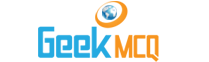Engineering :: Strength Of Materials

1.  In case of non-dilatent materials, the maximum value of Poisson's ratio is
 A. 0.25 B. 0.33 C. 0.47 D. 0.5 E. 0.6

2.  The shape of the Kem area for a rectangular section is
 A. Circle B. Square C. Rectangle D. Parallelogram E. Rhombus

3.  If Young's modulus of elasticity is determined for mild steel in tension and compression, the two values will have a ratio (Et/Er) of
 A. 1 B. 0.5 C. 1.2 D. 2

4.  The number of elastic constants for a completely anisotropic elastic material which follows Hooke's law, is
 A. 2 B. 3 C. 4 D. 21 E. 25

5.  A material which undergoes no deformation till its yield point is reached and then it flows at a constant stress is known as
 A. Elasto-plastic B. Plasto-electric C. Rigid-plastic D. Rigid-elastic

6.  Strain rosettes are generally used for
 A. Measurement of load B. Measurement of shear strain C. Measurement of resilience D. Measurement of proof stress E. Measurement of longitudinal strains

7.  The column with highest equivalent length has
 A. Both ends fixed B. Both ends hinged and pin jointed C. One end fixed other end hinged D. None of the above

 A. One that does not exist B. One that is dead C. One that can be neglected D. One that does not move E. One that remains constant.

9.  A live load is one that
 A. Remains constant B. Cannot be neglected C. Has lived and is no more in existence D. Varies with time E. Varies continuously

10.  Strain is
 A. The change in metals B. The change in shape and direction in metal C. The result of load application on a body D. The change of form produced in a piece by the action of load E. None of the above